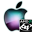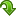ATOMS : Mathieu functions toolbox details

# Mathieu functions toolbox

Solve Mathieu equations, calculate Mathieu functions
Details
Version
3.0.2
A more recent valid version with binaries for Scilab 5.3 exists: 3.1
Authors
R. Coisson, G. Vernizzi, XK. Yang, N. Strelkov
Michael Baudin
Owner Organization
Parma University - DIGITEO - NRU MPEI
Maintainers
Nikolay Strelkov
Robert Coisson
Michael Baudin
Category
Creation Date
May 4, 2012
Source created on
Scilab 5.3.x
Binaries available on
Scilab 5.3.x:Windows 64-bitWindows 32-bitLinux 64-bitLinux 32-bitMacOSX
Install command
`--> atomsInstall("Mathieu")`
Report a bugDescription
```            Purpose
-------

This Mathieu Functions Toolbox is used to solve Mathieu function numerically
.

The Mathieu equation is a second-order homogeneous linear differential equation
and appears in several different situations in Physics: electromagnetic or
elastic wave equations with elliptical boundary conditions as in waveguides or
resonators, the motion of particles in alternated-gradient focussing or
electromagnetic traps, the inverted pendulum, parametric oscillators, the motion
of a quantum particle in a periodic potential, the eigenfunctions of the quantum
pendulum, are just few examples. Their solutions, known as Mathieu functions,
were first discussed by Mathieu in 1868 in the context of the free oscillations
of an elliptic membrane.

We present both the Floquet solution and solution in angular and radial
(modified) Mathieu functions [2-4].

The toolbox has 13 demos.
All demos can be acessed from "?->Scilab Demonstrations"

Features
--------
> Coordinate transformation
Almost all tasks, involving Mathieu functions have elliptical geometry. For
coordinate transformation we have functions:
* mathieu_cart2ell 	convert coordinates from Cartesian to elliptical
* mathieu_ell2cart 	convert coordinates from elliptical to Cartesian
* mathieu_cart2pol 	convert coordinates from Cartesian to polar
* mathieu_pol2cart 	convert coordinates from polar to Cartesian
* mathieu_ell_in_pol 	calculate polar coordinates of a point at known angle on
ellipse

For better understanding of elliptical coordinates you can use the demo named
"Elliptic and Cartesian coordinates".

> Floquet solution
For Floquet solution we have the following functions:
* mathieu_mathieuf 	evaluate characteristic values and expansion coefficients
* mathieu_mathieu 	evaluate periodic Mathieu functions by calling
mathieu_mathieuf
* mathieu_mathieuexp 	calculate the characteristic exponent of non-periodic
solutions and the coefficients of the expansion of the periodic factor
* mathieu_mathieuS 	calculate solutions of ME with arbitrary a and q
parameters (uses mathieu_mathieuexp)
* mathieu_elliptical 	calculte 3D plot of a mode with elliptical boundary
conditions

For Floquet solutions we have demos:
* Plots of ce_m(z,q), se_m(z,q) of order 0-5 for q = 1,10 for comparison with
Abramowitz and Stegun (Figs. 20.2-20.5, [3, pp. 725-726]).
* Tables of ce_m(z,q) and ce_m'(z,q) for comparison with Abramowitz and Stegun
(Table 20.1 [3, p. 748]).
* Tables of se_m(z,q) and se_m'(z,q) for comparison with Abramowitz and Stegun
(Table 20.1 [3, p. 749]).
* Plots of ce_m(z,q), se_m(z,q) of order 0-3 for q = 0-30 and z = [0, π]
(Fig. 2, [5, p. 235]).
* Comparison of 2 modes of elliptic membare with q = 0 and q = 8, even (see
).
* Comparison of 4 modes with q = 0 and q = 8, even & odd (see ).
* 3D plot of a mode with elliptical boundary conditions (see ).

> Solution in angular and radial (modified) functions
We present solutions of Mathieu equation as angular Mathieu functions [2,3]:
ce_m(z,q) and se_m(z,q) 
and solutions of modified Mathieu equation (2) as radial Mathieu functions [2,
3]:
Mc_m(1)(z,q), Ce_m(z,q), Mc_m(2)(z,q), Gey_m(z,q) and Ms_m(1)(z,q), Se_m(z,q),
Ms_m(2)(z,q), Fey_m(z,q).

Before calculating any Mathieu function we calculate expansion coefficients
and eigenvalues for given order m and parameter q using tri-diagonal matrixes
[5-8].

We have the following functions for computation solutions of Mathieu
equations:
* mathieu_Arm 	compute expansion coefficients 'Arm' and eigenvalue 'am' for
even angular and radial Mathieu functions
* mathieu_Brm 	compute expansion coefficients 'Brm' and eigenvalue 'bm' for
odd angular and radial Mathieu functions
* mathieu_ang_ce 	compute even angular Mathieu function 'ce' or its first
derivative
* mathieu_ang_se 	compute odd angular Mathieu function 'se' or its first
derivative
first derivative (kinds 1 and 2)
first derivative (kinds 1 and 2)
kind 'Ce' or its first derivative
kind 'Se' or its first derivative
second kind 'Fey' or its first derivative
second kind 'Gey' or its first derivative

During unit-testing all functions were tested against known tables:
eigenvalues, expansion coefficients and angular functions and its first
derivatives were compared with [3, 9], radial functions and their first
derivatives were compared with [9-12].

For eigenvalues we have demo, named "Stability chart for eigenvalues of
Mathieu`s equations" (for comparison with http://dlmf.nist.gov/28.17 ).

For modified solutions we have demos:
* Plots of Mc_m(1)(z,q), Ms_m(1)(z,q) of order 0-2 for q = 0-3 and z = [0,
π]
* Plots of Mc_m(2)(z,q), Ms_m(2)(z,q) of order 0-2 for q = 0-3 and z = [0,
π]
* Plots of Ce_m(z,q), Se_m(z,q) of order 0-2 for q = 0-3 and z = [0, π]
* Plots of Fey_m(z,q), Gey_m(z,q) of order 0-2 for q = 0-3 and z = [0, π]

All functions have examples with plots, some of them for comparison with [3,
4].

Bibliography
------------
1. R. Coïsson, G. Vernizzi and X.K. Yang, "Mathieu functions and numerical
solutions of the Mathieu equation", IEEE Proceedings of OSSC2009 (online at
http://www.fis.unipr.it/~coisson/Mathieu.pdf).
2. N.W. McLachlan, Theory and Application of Mathieu Functions, Oxford Univ.
Press, 1947.
3. M. Abramowitz and I.A. Stegun, Handbook of Mathematical Functions, Dover, New
York, 1965.
4. Chapter 28 Mathieu Functions and Hill's Equation. Digital Library of
Mathematical Functions. NIST. (online at http://dlmf.nist.gov/28).
5. J. C. Gutiérrez-Vega, R. M. Rodríguez-Dagnino, M. A. Meneses-Nava, and S.
Chávez-Cerda, "Mathieu functions, a visual approach", American
Journal of Physics, 71 (233), 233-242. An introduction to applications (online
at http://www.df.uba.ar/users/sgil/physics_paper_doc/papers_phys/modern/matheiu0.pdf).
6. J. J. Stamnes and B. Spjelkavik. New method for computing eigenfunctions
(Mathieu functions) for scattering by elliptical cylinders. Pure Appl. Opt. 4
251-62, 1995.
7. L. Chaos-Cador, E. Ley-Koo. Mathieu functions revisited: matrix evaluation
and generating functions. Revista Mexicana de Fisica, Vol. 48, p.67-75, 2002.
8. Julio C. Gutiérrez-Vega, "Formal analysis of the propagation of
invariant optical fields in elliptic coordinates", Ph. D. Thesis, INAOE,
México, 2000. (online at http://homepages.mty.itesm.mx/jgutierr/).
9. S. Zhang and J. Jin. Computation of Special Functions. New York, Wiley,
1996.
10. G. Blanch and D. S. Clemm. Tables relating to the radial Mathieu functions.
Volume 1. Functions of the First Kind. ARL, US Air Force. 1963. (online at http://catalog.hathitrust.org/Record/000585710).
11. G. Blanch and D. S. Clemm. Tables relating to the radial Mathieu functions.
Volume 2. Functions of the Second Kind. ARL, US Air Force. 1963. (online at http://catalog.hathitrust.org/Record/000585710).
12. E. T. Kirkpatrick. Tables of Values of the Modified Mathieu Functions.
Mathematics of Computation, Vol. 14, No. 70 (Apr., 1960), pp. 118-129. (online
at http://www.ams.org/journals/mcom/1960-14-070/S0025-5718-1960-0113288-4/S0025-5718-1960-0113288-4.pdf).            ```Files (2)Source code archive
```Bug fix release. Now ATOM will normally load on MacOS and Linux OS.
```OS-independent binary for Scilab 5.3.x
```Binary version
Automatically generated by the ATOMS compilation chain

```News (0)Comments (0)Leave a comment
You must register and log in before leaving a comment.Email notifications
Send me email when this toolbox has changes, new files or a new release.